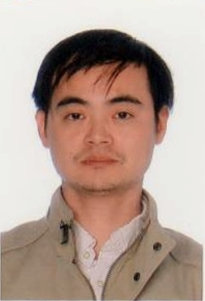Spectral Method for Maxwell Equations in Cole-Cole Dispersive Media
Speaker
Dr. Can Huang
Xiamen University
Abstract

In this talk, I shall consider time-dependent Maxwell equations in Cole-Cole dispersive media. The Cole-Cole model is the standard Maxwell equation coupled with a fractional time derivative term, which challenges the design and analysis of its numerical algorithm. By adopting the Matrix diagonalization method, the semidiscretisation of the model can be broken into a set of ordinary integro-differential equations (OIDE) with weakly singular kernel. Then, we propose an ansatz of solution for OIDEs. Using the ansatz, together with mapped numerical quadrature technique and well-conditioned matrix technique, we are able to produce an accurate approximation for OIDEs and thus, the original model as well.